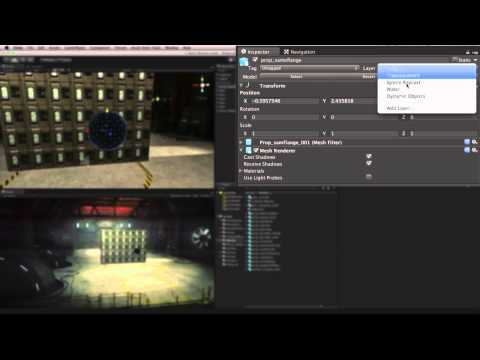# Brick Shooter

#### 难度: 新手

Create a wall of bricks and knock them down. In this Assignment you'll learn about Rigidbodies, Colliders, the Instantiate function and using Prefabs.## Brick Shooter

### Code snippet

``````using UnityEngine;
using System.Collections;

public class Shooter : MonoBehaviour
{
public Rigidbody projectile;
public Transform shotPos;
public float shotForce = 1000f;
public float moveSpeed = 10f;

void Update ()
{
float h = Input.GetAxis("Horizontal") * Time.deltaTime * moveSpeed;
float v = Input.GetAxis("Vertical") * Time.deltaTime * moveSpeed;

transform.Translate(new Vector3(h, v, 0f));

if(Input.GetButtonUp("Fire1"))
{
Rigidbody shot = Instantiate(projectile, shotPos.position, shotPos.rotation) as Rigidbody;
}
}
}``````
``````#pragma strict

public var projectile : Rigidbody;
public var shotPos : Transform;
public var shotForce : float = 1000f;
public var moveSpeed : float = 10f;

function Update ()
{
var h : float = Input.GetAxis("Horizontal") * Time.deltaTime * moveSpeed;
var v : float = Input.GetAxis("Vertical") * Time.deltaTime * moveSpeed;

transform.Translate(new Vector3(h, v, 0f));

if(Input.GetButtonUp("Fire1"))
{
var shot : Rigidbody = Instantiate(projectile, shotPos.position, shotPos.rotation);
}
}``````
``````import UnityEngine
import System.Collections

public class Shooter(MonoBehaviour):

public projectile as Rigidbody

public shotPos as Transform

public shotForce as single = 1000.0F

public moveSpeed as single = 10.0F

private def Update():
h as single = ((Input.GetAxis('Horizontal') * Time.deltaTime) * moveSpeed)
v as single = ((Input.GetAxis('Vertical') * Time.deltaTime) * moveSpeed)

transform.Translate(Vector3(h, v, 0.0F))

if Input.GetButtonUp('Fire1'):
shot = (Instantiate(projectile, shotPos.position, shotPos.rotation) as Rigidbody)